### 2016 SSC CGL Tier 2 Quantitative Aptitude 1 Dec Shift – 1 Question Paper with Answer Key

1. Let 0 < x < 1. Then die correct inequality is
1) x < √x < x2
2) √x < X < X2
3) x2< x < √x
4) √x < x2 < x

2. Three bells ring at interval of 36 seconds, 40 seconds and 48 seconds respectively. They start ringing together at a particular time. They will ring together after every
1) 6 minutes
2) 12 minutes
3) 18 minutes
4) 24 minutes

3. If the sum of the digits of a three digit number is subtracted from that number, then it will always be divisible by
1) 3 only
2) 9 only
3) both 3 and 9
4) all of 3,6 and 9

4. Which of the following is correct 7
1) 2/3 < 3/5 < 11/15
2) 3/5 < 2/3 < 11/15
3) 11/15 < 3/5 < 2/3
4) 3/5 < 11/15 < 2/3

5. The greater of the two numbers whose product is 900 and sum exceeds their difference by 30 is
1) 60
2) 75
3) 90
4) 100

6. The smallest fraction, which should be added to the sum of 2 ½, 3 1/3, 4 ¼ and 5 1/5 to make the result a whole number, is
1) 13/60
2) 1/4
3) 17/60
4) 43/60

7. Find the cube root of (-13824)
OR
Find the value of 3√-13824
1) 38
2) -38
3) 24
4) -24

8. The sum of three positive numbers is 18 and their product is 162. If the sum of two numbers is equal to the third then the sum of squares of the numbers is
1) 120
2) 126
3) 132
4) 138

9. The sum of three consecutive even numbers is 28 more than the average of these three numbers. Then the smallest of these three numbers is
1) 6
2) 12
3) 14
4) 16

10. In a division sum, the divisor ‘d’ is 10 times the quotient ‘q’ and 5 times the remainder ‘r’. If r = 46, the dividend will be
1) 5042
2) 5328
3) 5336
4) 4276

11. A man can do a piece of work in 30 hours. If he works with his son then the same piece of work is finished in 20 hours. If the son works alone he can do the work in
1) 60 hours
2) 50 hours
3) 25 hours
4) 10 hours

12. A water tap fills a tub in ‘p’ hours and a sink at the bottom empties it in q’ hours. If p < q and both tap and sink are open, the tank is filled in ‘r’ hours;
1) 1/r = 1/p+1/q
2) 1/r = 1/p-1/q
3) r = p+ q
4) r = p-q

13. John does 1/2 piece of work in 3 hours, Joe does 1/4 of the remaining work in 1 hour and George finishes remaining work in 5 hours. How long would it have taken the three working together to do the work ?
1) 2 1/7 hours
2) 3 1/7 hours
3) 3 8/11 hours
4) 2 8/11 hours

14. A does 2/5 of a work in 9 days. Then B joined him and they together completed the remaining work in 6 days. B alone can finish the whole work in
1) 6 12/13 days
2) 8 2/11 days
3) 10 days
4) 18 days

15. The daily wages of A and B respectively are Rs.3.50 and 2.50 . When A finishes a certain work, he gets a total wage of Rs. 63. When B does the same work, he gets a total wage Rs.75. If both of them do it together what is the cost of the work ?
1) Rs. 67.50
2) Rs. 27.50
3) RS. 60.50
4) Rs. 70.50

16. A man does double the work done by a boy in the same time. The number of days that 3 men and 4 boys will take to finish a work which can be done by 10 men in 8 days is
1) 4
2) 16
3) 7 3/11
4) 8 4/5

17. The marked price of an article is 30% higher than the cost price. If a trader sells the articles allowing 10% discount to customer, then the gain percent will be
1) 17
2) 20
3) 19
4) 15

18. A merchant marked the price of an article by increasing its production cost by 40%. Now he allows 20% discount and gets a profit of Rs. 48 after selling it The production cost is
1) Rs. 320
2) Rs. 360
3) Rs. 400
4) Rs. 440

19. A watch dealer pays 10% customs duty on a watch which costs Rs.500 abroad. He desires to make a profit of 20% after giving a discount of 25% to the buyer. The marked price should be
1) RS. 950
2) Rs. 800
3) Rs. 880
4) Rs. 660

20. A shopkeeper allows 20% discount on his advertised price and to make a profit of 25% on his outlay. What is the advertised price (in Rs.) on which he gains Rs. 6000 ?
1) 36000
2) 37500
3) 39000
4) 42500

21. Rs.2420 were divided among A, B, C so that A : B = 5 : 4 and B : C = 9 : 10 then C gets
1) 680
2) 800
3) 900
4) 950

22. 49 Kg of blended tea contain Assam and Darjeeling tea in the ratio 5 : 2. Then the quantity of Darjeeling tea is to be added to the mixture to make the ratio of Assam to Darjeeling tea 2 : 1 is
1) 4.5 Kg
2) 3.5 Kg
3) 5 kg
4) 6 kg

23. Among 132 examinees of a certain school, the ratio of successful to unsuccessful students is 9 : 2. Had 4 more students passed, then the ratio of successful to unsuccessful students will be
1) 14 : 3
2) 14 : 5
3) 28 : 3
4) 28 : 5

24. In a regiment the ratio between the number of officers to soldiers was 3:31 before battle. In a battle 6 officers and 22 soldiers were killed and the ratio became 1 : 13, the number of officers in the regiment before battle was
1) 31
2) 38
3) 21
4) 28

25. Three containers have their volumes in the ratio 3:4:5. They are full of mixtures of milk and water. The mixtures contain milk and water in the ratio of (4 :1), (3 : 1) and (5 : 2) respectively. The contents of all these three containers are poured into a fourth container. The ratio of milk and water in the fourth container is
1) 4:1
2) 151 : 48
3) 157 : 53
4) 5:2

26. In what proportion must a grocer mix sugar at Rs.12 a kg and Rs.7 a kg so as to make a mixture worth Rs.8 a kg ?
1) 7 : 12
2) 1:4
3) 2:3
4) 12 : 7

27. Fifteen movie theatres average 600 customers per theatre per day. If six of the theatres close down but the total theatre attendance stays the same, then the average daily attendance per theatre among the remaining theatres is
1) 900
2) 1000
3) 1100
4) 1200

28. The average weight of A, B and C is 45 kg. If the average weight of A and B be 40 kg and that of B and C be 43 kg, then the weight of B is:
1) 31kg
2) 32 kg
3) 29.5 kg
4) 35 kg

29. The batting average for 40 innings of a cricket player is 50 runs. His highest score exceeds his lowest score by 172 runs. If these two innings are excluded, the average of the remaining 38 innings is 48 runs. The highest score of the player is
1) 165
2) 170
3) 172
4) 174

30. The average of 7 consecutive numbers is 20. The largest of these numbers is
1) 20
2) 23
3) 24
4) 26

31. Mukesh has twice as much money as Soham. Soham has 50% more money than Pankaj. If the average money with them is Rs.110, then Mukesh has
1) 155
2) 160
3) 180
4) 175

32. The average daily income of 7 men, 11 women and 2 boys is Rs.257.50. If the average daily income of the men is Rs.10 more than that of women and the average daily income of the women is Rs.10 more than that of boys, the average daily income of a man is
1) Rs.277.5
2) Rs.250
3) Rs.265
4) Rs.257

33. If the profit on selling an article for Rs.425 is the same as the loss on selling it for Rs.355, then the cost price of the article is
1) Rs.410
2) Rs.380
3) Rs.400
4) Rs.390

34. A & B jointly made a profit of Rs.1650 and they decided to share it such that 1/3 of A’s profit is equal to 2/5 of B’s profit Then profit of B is
1) Rs.700
2) Rs.750
3) Rs.850
4) Rs.800

35. 4% of the selling price of an article is equal to 5% of its cost price. Again 20% of the selling price is Rs.120 more than 22% of its cost price. The ratio of cost price & selling price is
1) 2:3
2) 3:2
3) 4:5
4) 5:4

36. Due to 25% fall in the rate of eggs, one can buy 2 dozen eggs more than before by investing Rs.162. Then the original rate per dozen of the eggs is
1) Rs. 22
2) Rs.24
3) Rs. 27
4) Rs. 30

37. Last year Mr. A bought two paintings. This year he sold them for Rs. 20,000 each. On one, he made a 25% profit and on the other he had a 25% loss. Then his net profit or loss is
1) He lost more than Rs.2000
2) He lost less than Rs. 2000
3) He earned more than Rs. 2000
4) He earned less than Rs.2000

38. A shopkeeper sells rice at 10% profit and uses weight 30% less than the actual measure. His gain percent is
1) 57 2/3%
2) 57 1/7%
3) 57 2/5%
4) 57 3/7%

39. What % of a day is 30 minutes ?
1) 2.83
2) 2.083
3) 2.09
4) 2.075

40. A businessman’s earning increase by 25% in one year but decreases by 4% in the next Going by this pattern, after 5 years, his total earnings would be Rs.72000. What is his present earning?
1) Rs. 10000
2) Rs. 80000
3) Rs. 40000
4) Rs. 54000

41. In an examination 73% of the candidates passed in quantitative aptitude test, 70% passed in General awareness and 64% passed in both. If 6300 failed in both subjects the total number of examinees were
1) 60000
2) 50000
3) 30000
4) 25000

42. A man spends 75% of his income. His income increases by 20% and his expenditure also increases by 10%. Find the percentage increase in his savings.
1) 25%
2) 50%
3) 15%
4) 10%

43. On a river, Q is the mid-point between two points P and R on the same bank of the river. A boat can go from P to Q and back in 12 hours, and from P to R in 16 hours 40 min. How long would it take to go from R to P ?
1) 3 1/3 hr.
2) 5 hr.
3) 6 2/3 hr.
4) 7 1/3 hr.

44. A car can finish a certain journey in 10 hours at a speed of 42 kmph. In order to cover the same distance in 7 hours, the speed of the car (km/h) must be increased by:
1) 12
2) 15
3) 18
4) 24

45. A man travels 450 km to his home partly by train and partly by car. He takes 8 hrs 40 mins if he travels 240 km by train and rest by car. He takes 20 mins more if he travels 180 km by train and the rest by car. The speed of the car in km/hr is
1) 45
2) 50
3) 60
4) 48

46. A train ‘B’ speeding with 100 kmph crosses another train C, running in the same direction, in 2 mins. If the length of the train B and C be 150m and 250m respectively, what is the speed of the train C (in kmph) ?
1) 75
2) 88
3) 95
4) 110

47. The compound interest on Rs. 30,000 at 7% per annum for n years is Rs. 4347. The value of n is
1) 3
2) 2
3) 4
4) 5

48. If A borrowed Rs. P at x% and B borrowed Rs. Q (> P) at y% per annum at simple interest at the same time, then the amount of their debts will be equal after
1) 100(Q-P/P x-Q y) years
2) 100(P x-Q y/Q-P) years
3) 100(P x-Q y/P-Q) years
4) 100(P-Q/P x-Q y) years

49. A man invested a sum of money at compound interest. It amounted to Rs. 2420 in 2 years and to Rs. 2662 in 3 years. Find the sum.
1) Rs. 1000
2) Rs. 2000
3) Rs. 5082
4) Rs. 3000

50. If a sum of money becomes 4000 in 2 yrs and 5500 in 4 yrs 6 months at the same rate of simple interest per annum. Then the rate of simple interest is
1) 21 3/7%
2) 21 2/7%
3) 21 1/7%
4) 21 5/7%

51. A hollow cylindrical tube 20 cm long is made of iron and its external and internal diameters are 8 cm and 6 cm respectively. The volume (in cubic cm) of iron used in making the tube is (Take π = 22/7)
1) 1760
2) 440
3) 220
4) 880

52. If the areas of three adjacent faces of a rectangular box which meet in a corner are 12 cm2, 15 cm2 and 20 cm2 respectively. Then the volume of the box is
1) 3600 cm3
2) 300 cm3
3) 60 cm3
4) 180 cm3

53. The ratio between the length and the breadth of a rectangular park is 3 : 2. If a man cycling along the boundary of the park at the speed of 12 km/hour completes one round in 8 minutes, then the area of the park is
1) 153650 m2
2) 135600 m2
3) 153600 m2
4) 156300 m2

54. If the radius of a right circular cylinder open at both the ends, is decreased by 25% and the height of the cylinder is increased by 25%. Then the curved surface area of the cylinder thus formed
1) remains unaltered
2) is increased by 25%
3) is increased by 6.25%
4) is decreased by 6.25%

55. A cylindrical pencil of diameter 1.2 cm has one of its end sharpened into a conical shape of height 1.4 cm. The volume of the material removed is
1) 1.056 cm3
2) 4.224 cm3
3) 10.56 cm3
4) 42.24 cm3

56. A rectangular park 60 m long and 40 m wide has two concrete crossroads running in the middle of the park and rest of the park has been used as a lawn. If the area of the lawn is 2109 m2 then the width of the road is
1) 3 m
2) 5 m
3) 6 m
4) 2 m

57. Four circles of equal radii are described about the four corners of a square so that each touches two of the other circles. If each side of the square is 140 cm then area of the space enclosed between the circumference of the circle is (take π = 22/7)
1) 4200 cm2
2) 2100 cm2
3) 7000 cm2
4) 2800 cm2

58. The amount of concrete required to build a concrete cylindrical pillar whose base has a perimeter 8.8 metre and curved surface area 17.6 sq. metre, is (Take π = 22/7)
1) 8.325 m3
2) 9.725 m3
3) 10.5 m3
4) 12.32 m3

59. A hemispherical bowl of internal radius 9 cm, contains a liquid. This liquid is to be filled into small cylindrical bottles of diameter 3 cm and height 4 cm. Then the number of bottles necessary to empty the bowl is
1) 18
2) 45
3) 27
4) 54

60. A rectangular water tank is 80 m x 40 m. Water flows into it through a pipe of 40 sq.cm at the opening at a speed of 10 km/hr. The water level will rise in the tank in half an hour is
1) 3/2 cm
2) 4/9 cm
3) 5/9 cm
4) 5/8 cm

61. A square and a regular hexagon are drawn such that all the vertices of the square and the hexagon are on a circle of radius r cm. The ratio of area of the square and the hexagon is
1) 3 : 4
2) 4 : 3√3
3) √2 : √3
4) 1 : √2

62. A solid cylinder has the total surface area 231 sq. cm. If its curved surface area is 2/3 of the total surface area, then the volume of the cylinder is
1) 154 cu.cm
2) 308 cu.cm
3) 269.5 cu.cm
4) 370 cu.cm 370

63. The lateral surface area of frustum of a right circular cone, if the area of its base is 16п cm2 and the diameter of circular upper surface is 4 cm and slant height 6 cm, will be
1) 30 п cm2
2) 48 п cm2
3) 36 п cm2
4) 60 п cm2

64. The diameter of a sphere is twice the diameter of another sphere. The surface area of the first sphere is equal to the volume of the second sphere. The magnitude of the radius of the first sphere is
1) 12
2) 24
3) 16
4) 48

65. A right circular cylinder having diameter 21 cm & height 38 cm is full of ice cream. The ice cream is to be filled in cones of height 12 cm and diameter 7 cm having a hemispherical shape on the top. The number of such cones to be filled with ice cream is
1) 54
2) 44
3) 36
4) 24

66.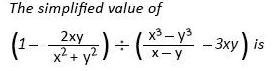1) 1/x2-y2
2) 1/x2+y2
3) 1/x-y
4) 1/x+y

67.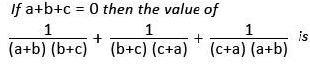1) 0
2) 1
3) 3
4) 2

68. Ifx2 + y2 + 2x+1 = 0, then the value of x31 + y35 is
1) -1
2) 0
3) 1
4) 2

69.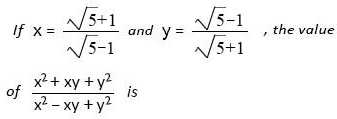1) 3/4
2) 4/3
3) 3/5
4) 5/3

70. IF (x-1/x)2 = 3, then the value of x6+1/x6 equals
1) 90
2) 100
3) 110
4) 120

71. If x4 + 2X3 + ax2 + bx + 9 is a perfect square, where a and b are positive real numbers, then the value of a and b are
1) a = 5, b = 6
2) a = 6, b = 7
3) a = 7, b = 6
4) a = 7, b = 8

72. If a2+b2+c2 = 16, x2+y2+z2 = 25 and ax+ by + cz = 20, then the value of a+ b+ c/x+ y+ z
1) 3/5
2) 5/3
3) 4/5
4) 5/4

73.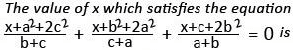1) (a2 + b2 + c2)
2) -(a2 + b2 + c2)
3) (a2 + 2b2 + c2)
4) -(a2 + b2 + 2c2)

74. If a3 = 117 + b3 and a = 3 + b, then the value of a + b is :
1) ±7
2) ±49
3) ± 13
4) 0

75. If a+ 1/a = -2 then the value of a1000 + a-1000 is
1) 2
2) 0
3) 1
4) 1/2

76. ΔABC is similar to ΔDFF. If area of ΔABC is 9 sq.cm. and area of ADEF is 16 sq.cm. and BC = 2.1 cm. then the length of EF will be
1) 5.6 cm
2) 2.8 cm
3) 3.7 cm
4) 1.4 cm

77. A chord of a circle is equal to its radius. The angle subtended by this chord at a point on the circumference is
1) 80°
2) 60°
3) 30°
4) 90°

78. Let two chords AB and AC of the larger circle touch the smaller cirde having same centre at X and Y. Then XY = ?
1) BC
2) ½ BC
3) 1/3 BC
4) ¼ BC

79. Let G be the centroid of the equilateral triangle ABC of perimeter 24 cm. Then the length of AG is
1) 2√3 cm
2) 8/√3 cm
3) 8√3 cm
4) 4√3 cm

80. A and B are the centres of two circles with radii 11 cm and 6 cm respectively. A common tangent touches these circles at P & Q respectively. IF AB = 13cm, then the length of PQ is
1) 13 cm
2) 17 cm
3) 8.5 cm
4) 12 cm

81. ABC is an isosceles triangle inscribed in a circle. If AB = AC = 12√5 and BC = 24 cm then radius of circle is ABC
1) 10 cm
2) 15 cm
3) 12 cm
4) 14 cm

82. ABC is an isosceles triangle where AB = AC which is circumscribed about a circle. If P is the point where the circle touches the side BC, then which of the following is true ?
1) BP = PC
2) BP > PC
3) BP < PC
4) BP = ½ PC

83. If D and E are the mid points of AB and AC respectively of ΔABC, then the ratio of the areas of ADE and BCED is ?
1) 1 : 2
2) 1 : 4
3) 2 : 3
4) 1 : 3

84. O is the circum centre of the isosceles ΔABC. Given that AB = AC = 17 cm and BC = 6 cm. The radius of the circle is
1) 3.015 cm
2) 3.205 cm
3) 3.025 cm
4) 3.125 cm

85. B1 is a point on the side AC of ΔABC and B1 B is joined. A line is drawn through A parallel to B1 B meeting BC at A1 and another line is drawn through C parallel to B1 B meeting AB produced at C1. Then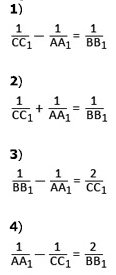86. The value of the expression (1 + sec22° + cot 68°)(1 – cosec22° + tan68°) is
1) 0
2) 1
3) -1
4) 2

87. If xsin3 Ө + ycos3 Ө = sin Ө cos Ө and x sin Ө – y cos Ө = 0, then the value of x2 + y2 equals
1) 1
2) ½
3) 3/2
4) 2

88. If sec Ө + tan Ө =m (>1), then the value of sin Ө is (0° < Ө < 90°)
1) 1-m2/1+m2
2) m2-1/m2+1
3) m2+1/m2-1
4) 1+m2/1-m2

89. If (a2 – b2)sin Ө + 2abcos Ө = a2 + b2, then tan Ө =
1) 2ab/a2-b2
2) a2-b2/2ab
3) ab/a2-b2
4) a2-b2/ab

90. A person from the top of a hill observes a vehide moving towards him at a uniform speed. It takes 10 minutes for the angle of depression to change from 45° to 60°. After this the time required by the vehicle to reach the bottom of the hill is
1) 12 min 20 sec
2) 13 min
3) 13 min 40 sec
4) 14 min 24 sec

91. If 2ycos Ө = x sin Ө and 2xsec Ө – y cosec Ө = 3, then the value of x2 + 4y2 is
1) 1
2) 2
3) 3
4) 4

92. From the top of a diff 100 metre high, the angles of depression of the top and bottom of a tower are 45° and 60° respectively. The height of the tower is
1) 100/3(3-√3)metre
2) 100/3(√3-1)metre
3) 100/3(2√3-1)metre
4) 100/3(√3-√2)metre

93. A vertical tower stands on a horizontal plane and is surmounted by a vertical flag staff of height h. At a point on the plane, the angle of elevation of the bottom of the flag staff is α and that of the top of the flag staff is β. Then the height of the tower is
1) h tan α
2) h tan α/tan β –tan α
3) h tan α/tan α –tan β
4) none of these

94. A man on the top of a tower, standing on the sea-shore, finds that a boat coming towards him takes 10 minutes for the angle of depression to change from 30° to 60°. How soon the boat reach the sea-shore ?
1) 5 minutes
2) 7 minutes
3) 10 minutes
4) 15 minutes

95. The expression of cotӨ+cosecӨ-1/cotӨ+cosecӨ+1 is equal to
1) 1+cosӨ/sin Ө
2) 1-cosӨ/sin Ө
3) cotӨ+1/cosec Ө
4) cotӨ-1/cosec Ө

96. The following pie-chart shows the monthly expenditure of a family on various items. If the family spends Rs. 825 on clothing, answer the question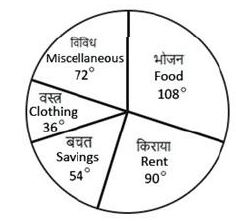What is the total monthly income of the family ?
1) Rs. 8025
2) Rs. 8250
3) Rs. 8520
4) Rs. 8052

97. The following pie-chart shows the monthly expenditure of a family on various items. If the family spends Rs. 825 on clothing, answer the questionWhat percent of the total income does the family save ?
1) 15%
2) 50%
3) 20%
4) 25%

98. The following pie-chart shows the monthly expenditure of a family on various items. If the family spends Rs. 825 on clothing, answer the questionWhat is the ratio of expenses on food and miscellaneous?
1) 3:4
2) 2:3
3) 3 : 2
4) 2 : 5

99. The following pie-chart shows the monthly expenditure of a family on various items. If the family spends Rs. 825 on clothing, answer the questionWhat is the average of expenses on clothing and rent?
1) Rs. 1443.75
2) Rs. 1344.57
3) Rs. 1574.34
4) Rs. 1734.45

100. The following pie-chart shows the monthly expenditure of a family on various items. If the family spends Rs. 825 on clothing, answer the questionThe ratio of average of expenses on food, clothing and miscellaneous items to the average of expenses on savings and rent is
1) 3:2
2) 1:3
3) 2 : 1
4) 1 : 1

1. C
2. B
3. C
4. B
5. A
6. D
7. D
8. B
9. B
10. C
11. A
12. B
13. C
14. D
15. A
16. B
17. A
18. C
19. C
20. B
21. B
22. B
23. D
24. C
25. C
26. B
27. B
28. A
29. D
30. B
31. C
32. C
33. D
34. B
35. C
36. C
37. A
38. B
39. B
40. C
41. C
42. B
43. D
44. C
45. A
46. B
47. B
48. A
49. B
50. A
51. B
52. C
53. C
54. D
55. A
56. A
57. A
58. D
59. D
60. D
61. B
62. C
63. C
64. B
65. A
66. B
67. A
68. A
69. B
70. C
71. C
72. C
73. B
74. A
75. A
76. B
77. C
78. B
79. B
80. D
81. B
82. A
83. D
84. D
85. B
86. D
87. A
88. B
89. B
90. C
91. D
92. A
93. B
94. A
95. A
96. B
97. A
98. C
99. A
100. D